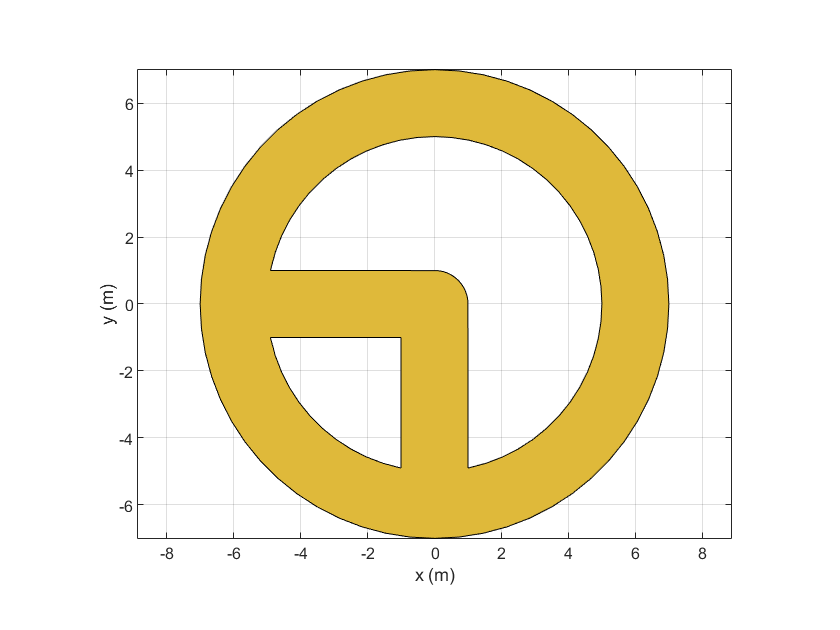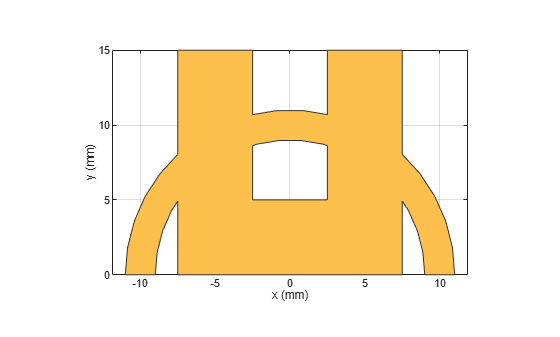plus

Shape1 + Shape2 for RF PCB shapes

Description

example

c = plus(shape1,shape2) calls the syntax shape1 + shape2 to unite two shapes.

Examples

collapse all

Create a curved bend shape with a length of 5 m

bend = bendCurved(Length=[5 5]);

Create an annular ring shape with the default inner radius of 5 m.

ring = ringAnnular;

Add the two shapes and display the result.

shapeSum = plus(bend,ring)
shapeSum =
Polygon with properties:

Name: 'mypolygon'
Vertices: [129x3 double]

show(shapeSum)Create the default curve shape.

shape1 = curve;

Create a right angle U-bend shape with an adjusted size and position to complement the curve shape.

shape2 = ubendRightAngle(Length=[5 18 5],ReferencePoint=[0 -5]);

Add the two shapes using the + operator, and display the resulting Polygon object.

shapeSum = shape1+shape2
shapeSum =
Polygon with properties:

Name: 'mypolygon'
Vertices: [91x3 double]

show(shapeSum)Input Arguments

collapse all

First shape created using custom elements and shape objects of RF PCB Toolbox™, specified as an object.

Example: shape1 = bendCurved; specifies the first shape as a bendCurved object.

Second shape created using custom elements and shape objects of RF PCB Toolbox, specified as an object.

Example: shape2 = ringAnnular; specifies the second shape as a ringAnnular object.